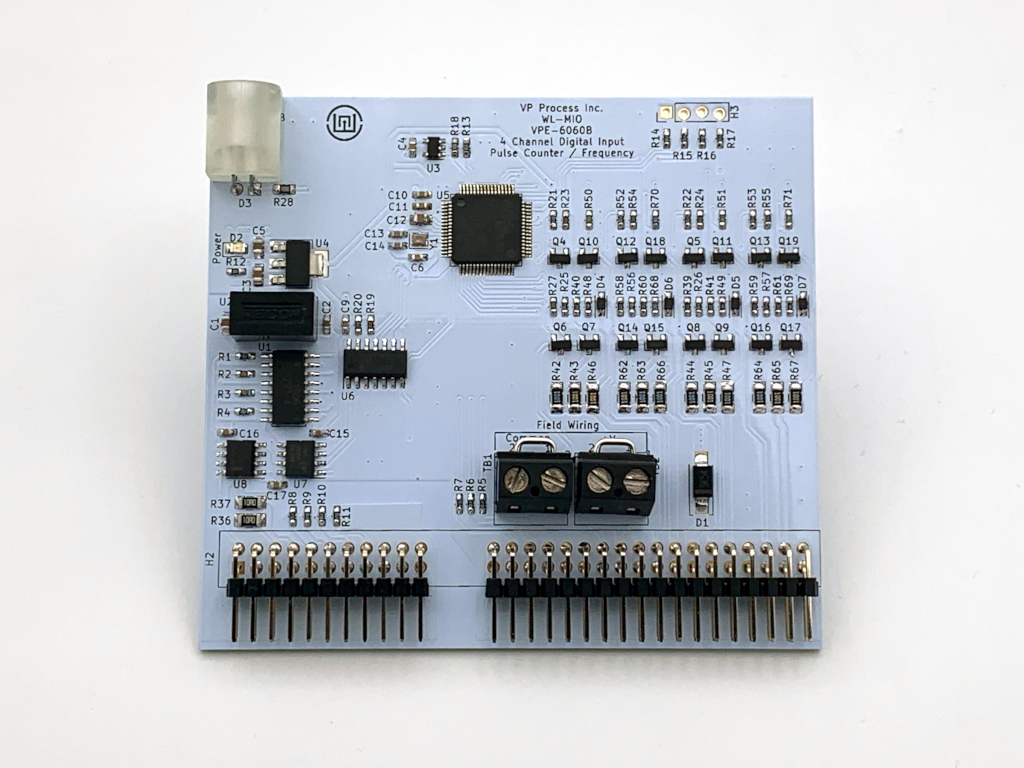### Application Note: WL-MIO-AN-36060

#### 4 Channel Digital Input Module - Discrete Input, Frequency Input or Pulse Counter InputThe VPE-6060 Digital Input 4 Channel Module has 4 inputs that can be independantly read and configured. Each input channel can be configured as discrete input ON/OFF, frequency, or Pulse Counter.
The "sample inrterval" can be programmed for this module, and is factory defauilted to 500 mS.
For this module, this value acts as the "gate time" for frequency and pulse counter modes.
It is global for all four channels in this version.

For the each channel, the following configuration values can be programmed:

• channel.bias
Used to control pull-up/pull-down on this channel
0 is no bias, 1 is pulldown, 2 is pullup (NPN or PNP)
• channel.mode
Channel input mode:
0 for "basic" mode (simple 0 or 1 reading)
1 for frequency input
2 for pulse counter
• channel.polarity
For pulse counter/frequency modes this determines whether to listen on falling/rising edge
0 for rising edge
1 for falling edge
• channel.input
Final input value. When in pulse counter mode the internal counter is reset upon register access.

The module can be installed in any backplane position. The NodeID referred to in the demo program is the "Backplane ID" determined by the backplane jumpers and the addition of the slot in which the power supply is plugged into.
Example 1: If the backplane has no jumpers and the analog input module is plugged into slot position 0, the NodeID is 0 + 0 = 0.
Example 2: If the backplane has a jumper in the "8" position and the analog input module is plugged into slot position 2, the NodeID is 8 + 2 = 10.

During normal operation, the status LED flashes GREEN once per second as a "heartbeat" indicator that everything is functioning normally. If the onboard firmware detects any fault conditions, the Status LED will flash either AMBER or RED.

For the following demo code to work, the "libwlmio" library has to be installed.

#### Demo Python Code

The Backplane has ID = 0 and the analog input module is plugged into slot postion 6.

```# vpe6060 Digital Input 4 Channel Demo Program
# Configure Channels as Discrete ON/OFF Inputs

import asyncio
from pywlmio import *

NodeID = 6  #NodeID location is the Bacplane ID (Jumpers) and Power Supply Slot location

async def main():
init()

di = VPE6060(NodeID)

try:
await asyncio.gather(
di.ch1.configure(0,0,0),   # mode = 0, polarity = 0, bias = 0
di.ch2.configure(0,0,0),   # mode = 0, polarity = 0, bias = 0
di.ch3.configure(0,0,0),   # mode = 0, polarity = 0, bias = 0
di.ch4.configure(0,0,0)    # mode = 0, polarity = 0, bias = 0
)

except WlmioWrongNodeError:
print("Error NodeID = %d Wrong module installed" % NodeID)  # Error Check if wrong type of module installed
except WlmioInternalError:
print("Error NodeID = %d Timed out" % NodeID)               # Error Check - Typically module not installed

while True:
try:
a = await asyncio.gather(
)

print("Module VPE6060 NodeID = %d" % NodeID)
print("Reading Array = ", a)  # Array holds all input channel readings
print("DI Input 1 = " , a, " 1=ON, 0=OFF")
print("DI Input 2 = " , a, " 1=ON, 0=OFF")
print("DI Input 3 = " , a, " 1=ON, 0=OFF")
print("DI Input 4 = " , a, " 1=ON, 0=OFF")
print("")

except WlmioWrongNodeError:
print("Error NodeID = %d Wrong module installed" % NodeID)  # Error Check if wrong type of module installed
except WlmioInternalError:
print("Error NodeID = %d Timed out" % NodeID)               # Error Check - Typically module not installed

await asyncio.sleep(1)

asyncio.run(main(), debug=True)
```

Output from the demo program in a terminal window:

```pi@raspberrypi:~/Desktop/wlmio/wlmio_rpi/src/demos \$ python3 vpe6060_demo.py
Module VPE6060 NodeID = 6
Reading Array =  [1, 0, 1, 0]
DI Input 1 =  1  1=ON, 0=OFF
DI Input 2 =  0  1=ON, 0=OFF
DI Input 3 =  1  1=ON, 0=OFF
DI Input 4 =  0  1=ON, 0=OFF
```